## Series Solutions: Hermite's Equation#### Exercise 1:

Find the Hermite Polynomials of order 1 and 3.

Recall that the recurrence relations are given by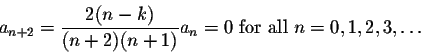We have to evaluate these coefficients for k=1 and k=3, with initial conditions a0=0, a1=1.

When k=1,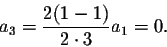Consequently all odd coefficients other than a1 will be zero. Since a0=0, all even coefficients will be zero, too. Thus

H1(t)=t.

When k=3,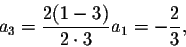and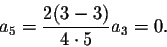Consequently all odd coefficients other than a1 and a3 will be zero. Since a0=0, all even coefficients will be zero, too. Thus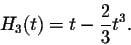[Back] [Exercises] [Next]
[Algebra] [Trigonometry] [Complex Variables]
[Calculus] [Differential Equations] [Matrix Algebra]S.O.S MATHematics home page

Do you need more help? Please post your question on our S.O.S. Mathematics CyberBoard.Helmut Knaust
1998-07-08